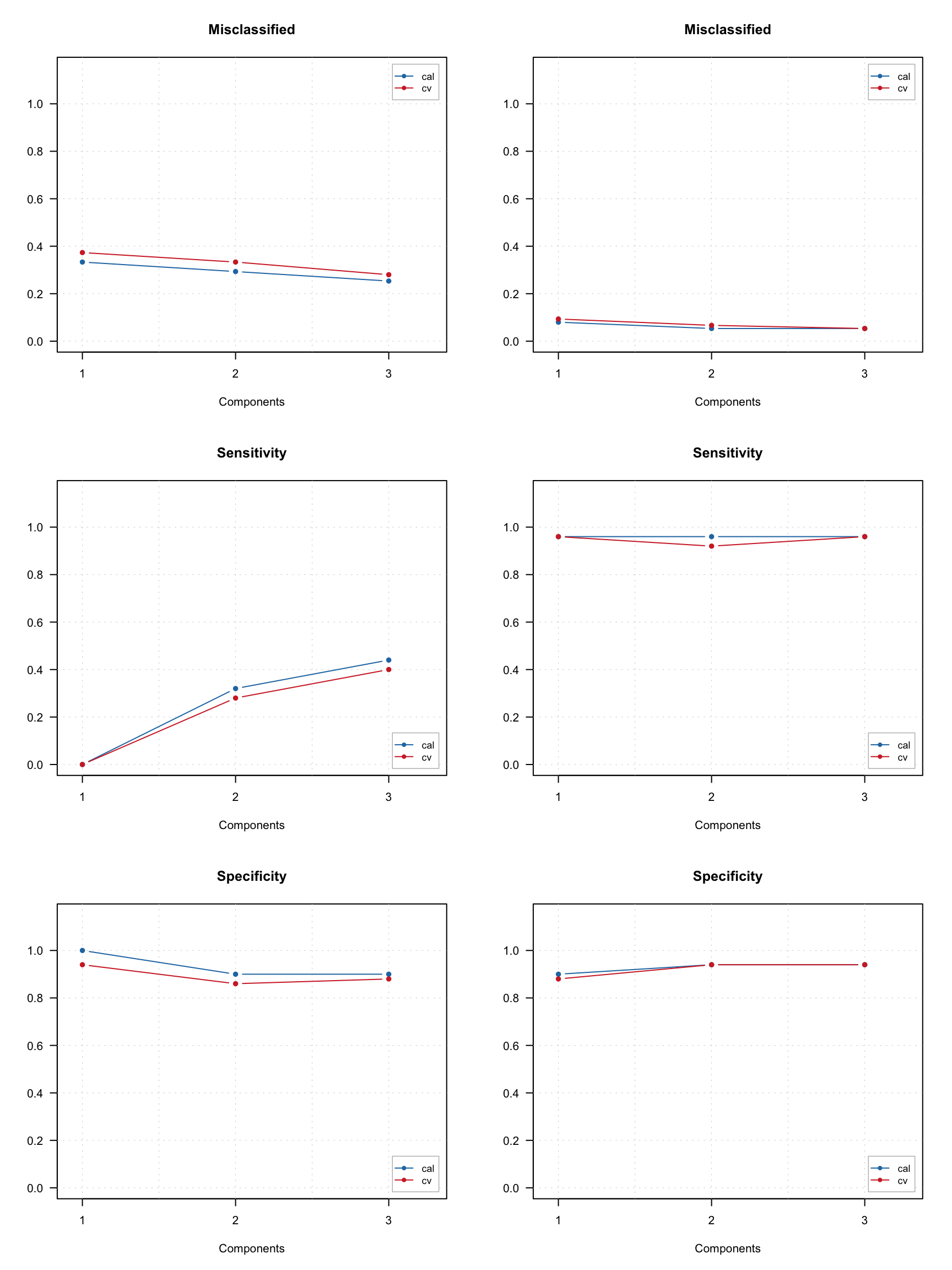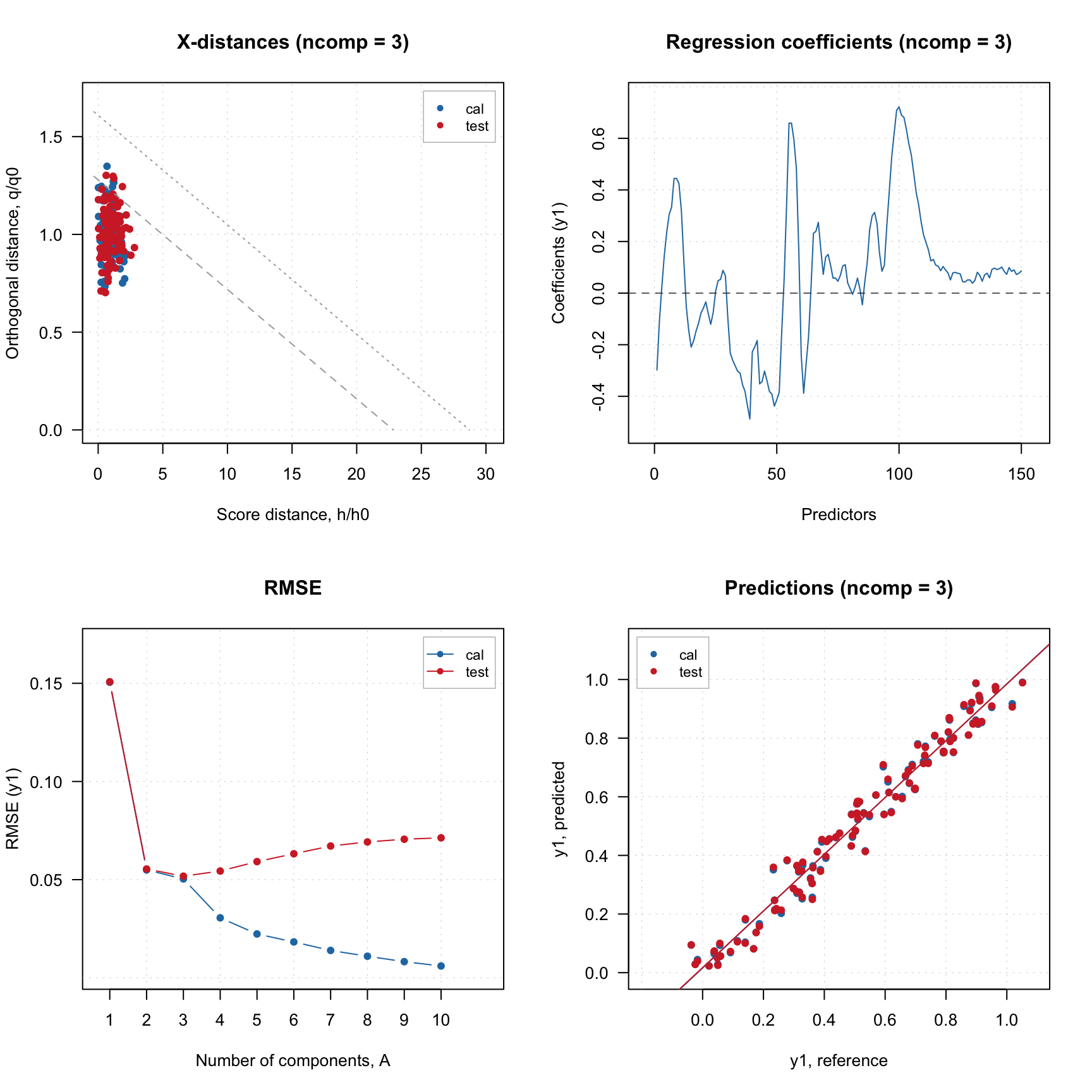## Performance plots

As in SIMCA you can show how sensitivity, specificity and total amount of misclassified samples depending on number of components by using corresponding plots. In case of multiple-classes model you can also provide a class number to show the plot for (by default package will show the plot for overall statistic computed for all classes).

par(mfrow = c(3, 2))
plotMisclassified(m.all, nc = 2)
plotMisclassified(m.vir)
plotSensitivity(m.all, nc = 2)
plotSensitivity(m.vir)
plotSpecificity(m.all, nc = 2)
plotSpecificity(m.vir)As usual, you can also change type of plot to line or scatter-line, change colors, etc. All PLS regression plots, including RMSE, X and Y variance, etc. will also work smoothly with PLS-DA models or results. You can also show regression coefficients like in the example below. To show regression coefficients for particular class you need to provide its number using parameter ny (the reason we use ny and not nc here is that this plot is inherited from PLS model). So in our example both plots show regression coefficients for prediction of virginica objects (left obtained using multiple class model and right — using one class model).

par(mfrow = c(1, 2))
plotRegcoeffs(m.all, ncomp = 3, ny = 3)
plotRegcoeffs(m.vir, ncomp = 1, show.ci = TRUE)